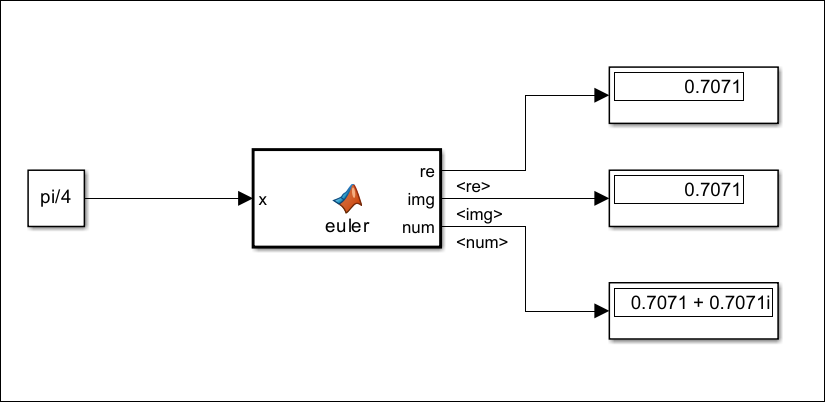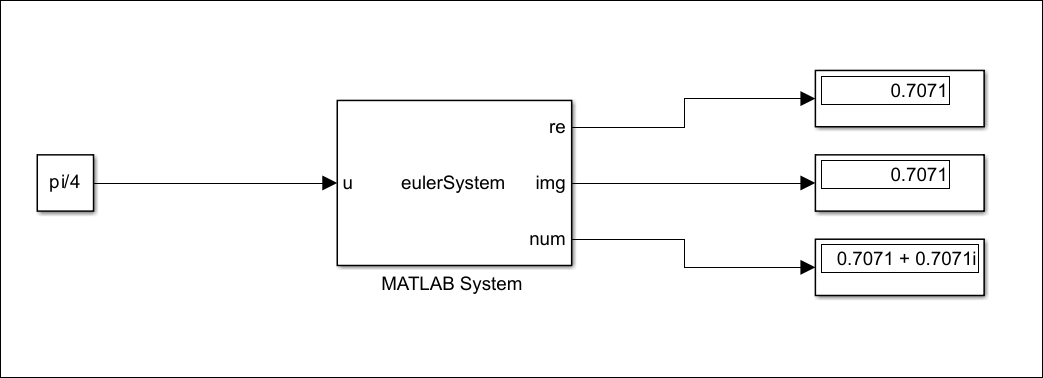## Overview of Integrating Python Code with Simulink

This topic provides an overview of integrating Python® code with Simulink®. You can set up your system to use Python with Simulink and use the MATLAB Function block or the MATLAB System block to integrate Python code with Simulink.

### Configure System to Use Python

To call Python modules in MATLAB®, you must have a compatible version of Python installed. For more information on supported Python versions and setting up your system to use Python in MATLAB, see Configure Your System to Use Python.

You can access all standard Python libraries, third-party functionality, or user-created modules from MATLAB. For more information on using Python in MATLAB, see Access Python Modules from MATLAB - Getting Started.

### Integrate Python Code with Simulink Using MATLAB Function Block

Use the MATLAB Function block to integrate your simple Python code with Simulink. Call Python modules in the MATLAB Function block using `py.` as prefix for the Python function or class name.

This code implements a MATLAB Function that calculates the Euler formula for complex numbers for the provided input, using `py.math.cos` and `py.math.sin`.

```function [re, img, num] = euler(x) % Declare py.math.cos and py.math.sin as extrinsic functions coder.extrinsic('py.math.cos','py.math.sin'); % Preallocate outputs re = double(0); img = double(0); re = py.math.cos(x); img = py.math.sin(x); num = re + 1j*img; end```

• Python modules are not supported for code generation and require the MATLAB engine for execution. You can exclude Python calls from code generation and use the MATLAB engine for execution by declaring it as an extrinsic function using `coder.extrinsic` function. This code declares `py.math.cos` and `py.math.sin` as extrinsic functions.

`coder.extrinsic('py.math.cos','py.math.sin');`
• The output that an extrinsic function returns at runtime is an `mxArray`, also known as MATLAB array. The valid operations for a MATLAB array are limited to storing it in a variable, passing it to another extrinsic function, or returning it to MATLAB. To perform any other operation on an `mxArray` value, such as using it in an expression in your code, you must convert the `mxArray` to a known type at run time. To perform this action, assign the `mxArray` to a variable whose type is already defined by a prior assignment. For more details on preallocating `mxArray`, see Return Output of Extrinsic Function to MATLAB at Run Time. This code preallocates the datatype of the real and imaginary part of the number as a `double`.

```re = double(0); img = double(0);```

Implement the MATLAB Function in Simulink to calculate the Euler formula for an input.### Integrate Python Code with Simulink Using MATLAB System Block

You can use the MATLAB System block to integrate Python code with Simulink. Use this block when the algorithm requires handling of state dynamics, streaming data, or additional block customizations. You can call Python modules using `py.` as a prefix for the Python function or class name. A Python function call within the MATLAB System block does not need to be declared as an extrinsic function. Change the Simulate Using block parameter to ```Interpreted Excecution```.

This code implements a MATLAB System object that calls `py.math.cos` and `py.math.sin` to calculate the Euler formula for complex numbers.

```classdef eulerSystem < matlab.System % System object to calculate Euler's formula for an input methods (Access = protected) function [re, img, num] = stepImpl(obj, u) % Implement algorithm re = py.math.cos(u); img = py.math.sin(u); num = py.math.cos(u) + 1j*py.math.sin(u); end function [c1, c2, c3] = isOutputComplexImpl(obj) % Implement propagator to declare complexity of outputs c1 = false; c2 = false; c3 = true; end function [flag1, flag2, flag3] = isOutputFixedSizeImpl(obj) % Implement propagator to declare if outputs are fixed size flag1 = true; flag2 = true; flag3 = true; end function [sz_1, sz_2, sz_3] = getOutputSizeImpl(obj) % Implement propagator to declare output sizes sz_1 = [1,1]; sz_2 = [1,1]; sz_3 = [1,1]; end function [out_1, out_2, out_3] = getOutputDataTypeImpl(obj) % Implement propagator to declare output data types out_1 = "double"; out_2 = "double"; out_3 = "double"; end end end ```

Implement the System object™ in Simulink as shown below to calculate the Euler formula for an input.You should define propagator methods when output specifications for the system block cannot be inferred directly from inputs during model compilation. The code above implements the `isOutputComplexImpl`, `isOutputFixedSizeImpl`, `getOutputSizeImpl`, and `getOutputDataTypeImpl` propagator methods. For more information on propagation methods, see Add and Implement Propagation Methods.